## Excel BITOR function

The BITOR function returns the bitwise ‘OR’ of two given numbers.

Note: This function is only available in Excel 2013 and later versions.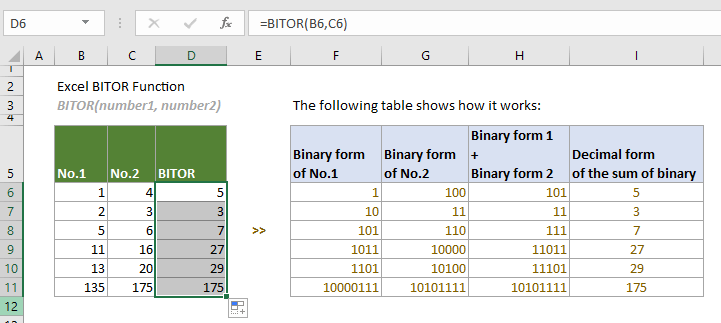#### Syntax

BITOR(number1, number2)

#### Arguments

• Number1 (required): This argument must be in decimal form greater than or equal to 0;
• Number2 (required): This argument must be in decimal form greater than or equal to 0.

#### Remarks

1. Arguments can be either of:
-- Numbers;
-- Cell references that contain numbers.
2. The #NUM! error value occurs if either of the following conditions is met:
-- Any of the supplied numbers is less than 0;
-- Any of the supplied numbers is greater than (2^48)-1.
3. The #VALUE! error value occurs if either of the supplied numbers is non-numeric.
4. In the result, if any bit of the parameter is 1 in that bit position, the bit will be 1.
-- For example, to calculate BITOR (13,20). The binary form of 13 is 1101, and the binary form of 20 is 10100. These two binary representations will be added as follows:
Tips:
As these two binaries have different digits, to help with comparison, 1101 can be considered as 01101.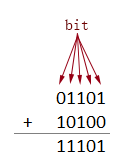#### Return value

It returns a numeric value.

#### Example

To calculate the bitwise ‘OR’ of the given numbers in the table below, you can do as follows.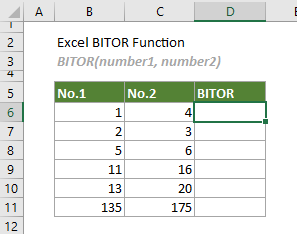Select a cell (says D6 in this case), enter the formula below and press Enter to get the bitwise ‘OR’ of the first two numbers. Select this result cell and then drag its AutoFill Handle down to get other results.

=BITOR(B6,C6)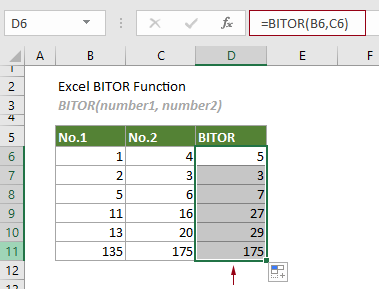Notes:

1) Arguments in the formula above are supplied as cell references that contain numbers. However, you can change the formula as follows:

=BITOR(1,4)

2) How does this BITOR function work?

Take the formula in D6 as an example: =BITOR(B6,C6)

2.1) First, the function converts the decimal number to binary.
In this case, the binary form of the decimal number ‘1’ is 1, and the binary form of the decimal number ‘4’ is 100.
Tips: You can apply the DEC2BIN function to easily convert a decimal number to binary in Excel.
2.2) Then the function adds these two binary representations.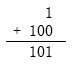In this case, the sum of these two binaries is 101.
2.3) Finally the function converts the binary number back to a decimal form.
In this case, the binary number 101 is returned in decimal form as an integer number 5.
Tips: You can apply the BIN2DEC function to easily convert a binary to decimal in Excel.

The following table shows how this function works in details.#### Related Functions

Excel BITRSHIFT function
The BITRSHIFT function returns the given number shifted right by the specified number of bits.

Excel BITXOR function
The BITXOR function returns the bitwise ‘XOR’ of two given numbers.

Excel BITLSHIFT Function
The BITLSHIFT function returns a decimal number shifted left by a specified number of bits.

Excel BITAND function
The BITAND function returns a decimal number representing the bitwise 'AND' of two supplied numbers.

### The Best Office Productivity Tools

#### Kutools for Excel - Helps You To Stand Out From Crowd

 Popular Features: Find, Highlight or Identify Duplicates  |  Delete Blank Rows  |  Combine Columns or Cells without Losing Data  |  Round without Formula ... Super VLookup: Multiple Criteria  |  Multiple Value  |  Across Multi-Sheets  |  Fuzzy Lookup... Adv. Drop-down List: Easy Drop Down List  |  Dependent Drop Down List  |  Multi-select Drop Down List... Column Manager: Add a Specific Number of Columns  |  Move Columns  |  Toggle Visibility Status of Hidden Columns  |  Compare Columns to Select Same & Different Cells ... Featured Features: Grid Focus  |  Design View  |  Big Formula Bar  |  Workbook & Sheet Manager | Resource Library (Auto Text)  |  Date Picker  |  Combine Worksheets  |  Encrypt/Decrypt Cells  |  Send Emails by List  |  Super Filter  |  Special Filter (filter bold/italic/strikethrough...) ... Top 15 Toolsets:  12 Text Tools (Add Text, Remove Characters ...)  |  50+ Chart Types (Gantt Chart ...)  |  40+ Practical Formulas (Calculate age based on birthday ...)  |  19 Insertion Tools (Insert QR Code, Insert Picture from Path ...)  |  12 Conversion Tools (Numbers to Words, Currency Conversion ...)  |  7 Merge & Split Tools (Advanced Combine Rows, Split Excel Cells ...)  |  ... and more

Kutools for Excel Boasts Over 300 Features, Ensuring That What You Need is Just A Click Away...#### Office Tab - Enable Tabbed Reading and Editing in Microsoft Office (include Excel)

• One second to switch between dozens of open documents!
• Reduce hundreds of mouse clicks for you every day, say goodbye to mouse hand.
• Increases your productivity by 50% when viewing and editing multiple documents.
• Brings Efficient Tabs to Office (include Excel), Just Like Chrome, Edge and Firefox.